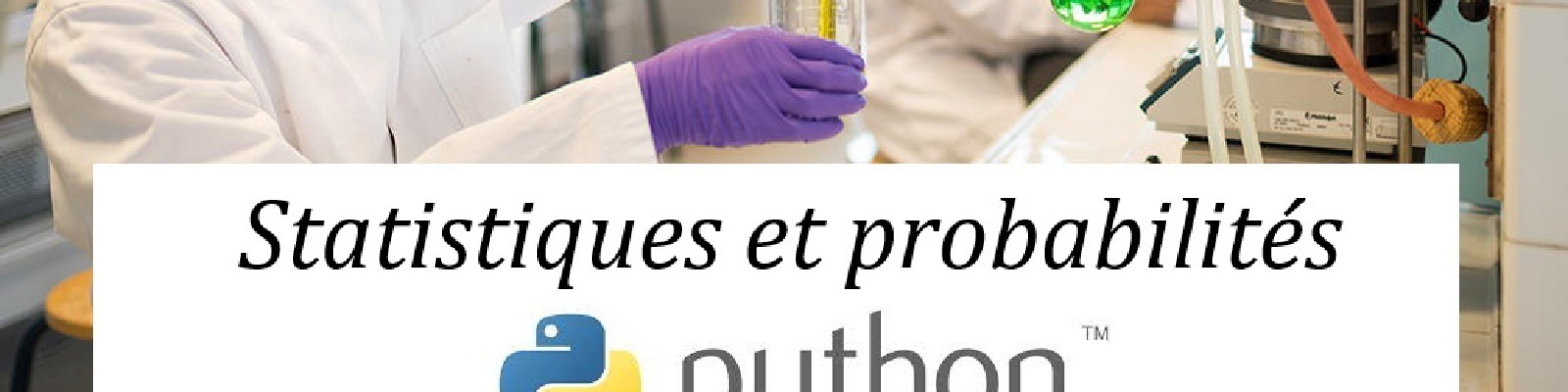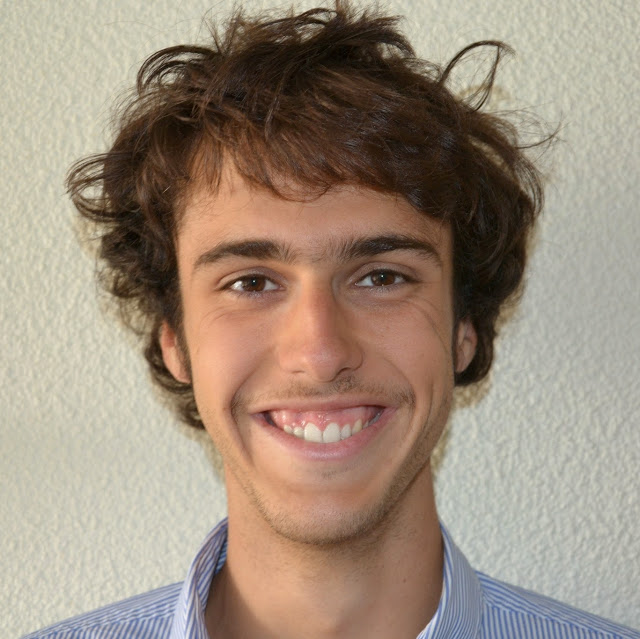Student in bio-chemistry courses © Arthur Pequin

# Overview

The Health and Science is established since 2107 and proposes to the students of second year medical studies, pharmacology and odontology an early formation to research.

# Course

• Introduction to statistical language and notions such as event, incompatibility or independancy.

• Enumerations and Bayes formula

• Classical estimators (mean, variance)

• Usual discret and continuous laws.

• An introduction to the statistical tests.

# Practical work on Python

• Statistical tests and multiplicity: Unitary tests, boxplot visualisations, Bonferroni and Benjamini-Hochberg corrections

• Linear regression: Python linear regression tools

• Newton-Raphson algorithm for likelihood maximization: Study of this algorithm for a real valued function depending on 2 variables